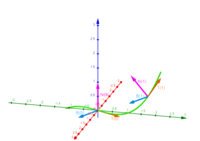# Solving cross product

#### burt

##### Junior Member
Maybe geogebra didn't actually indicate that the 'zero vector' <0, 0, 0> itself is undefined (because it is). Instead, I'd expect the message meant we can't plot a zero vector as an arrow.

The zero vector is a special case; it's defined as a vector having no direction. Any vector whose components are all zero has no magnitude. Without any length, an arrow can't point toward anything.

The scalar number zero is known as "the additive identity" because the sum of adding 0 to a Real number is the same Real number. That's a useful identity, in algebra. A zero vector is like "the vector-additive identity" because adding a zero vector to another vector doesn't change anything. That's useful in vector arithmetic.

That's not what you wrote in post #5. Each of the six vectors in post #5 have a defined magnitude and direction. None of them are zero vectors.Right, but I think I made a mistake in post #5. Unless you are telling me that my post #5 is correct and now I am wrong (which is very possible).

#### Otis

##### Senior Member
… I think I made a mistake in post #5. Unless you are telling me that my post #5 is correct …
Hi Burt. I mentioned in post #11 that I did not check your work in post #5. I think Jomo said that he'd checked B(0) and B(1).

Did you check your work? Is that why your changed some vectors? I can see only what you post.#### burt

##### Junior Member
Hi Burt. I mentioned in post #11 that I did not check your work in post #5. I think Jomo said that he'd checked B(0) and B(1).

Did you check your work? Is that why your changed some vectors? I can see only what you post.Meaning you can't see the images?

#### burt

##### Junior Member
Hi Burt. I mentioned in post #11 that I did not check your work in post #5. I think Jomo said that he'd checked B(0) and B(1).

Did you check your work? Is that why your changed some vectors? I can see only what you post.Here is my work - it's in mathJax from word. I can try to make it so that you can see it - I'm not sure if I will be able to.

) r\left(t\right)=<t,2t,t^3>\bigmr^\prime\left(t\right)=<1,2,3t^2>\bigm\left|\left|r^\prime\left(t\right)\right|\right|=\sqrt{1+4+9t^4}=\sqrt{5+9t^4}\bigmT\left(t\right)=<\frac{1}{\sqrt{5+9t^4}},\frac{2}{\sqrt{5+9t^4}},\frac{3t^2}{\sqrt{5+9t^4}}>\bigmT\left(0\right)=<\frac{1}{\sqrt5},\frac{2}{\sqrt5},0>\mathrm{and\mathrm{\ }}T\left(1\right)=<\frac{1}{\sqrt{14}},\frac{2}{\sqrt{14}},\frac{3}{\sqrt{14}}>\bigmT^\prime\left(t\right)=<\frac{-18t^3}{(9t^4+5)^\frac{3}{2}},\frac{-36t^3}{(9t^4+5)^\frac{3}{2}},\frac{30t}{(9t^4+5)^\frac{3}{2}}>\bigm\left|\left|T^\prime\left(t\right)\right|\right|=\sqrt{(\frac{-18t^3}{(9t^4+5)^\frac{3}{2}})^2+(\frac{-36t^3}{(9t^4+5)^\frac{3}{2}})^2+(\frac{30t}{(9t^4+5)^\frac{3}{2}})^2}=\frac{6\sqrt5t}{9t^4+5}\bigmN\left(t\right)=<\frac{-3\sqrt5t^2}{5\sqrt{9t^4+5}},\frac{-6\sqrt5t^2}{5\sqrt{9t^4+5}},\frac{\sqrt5}{\sqrt{9t^4+5}}>\bigmN\left(0\right)=\mathrm{undefined}\ \mathrm{and\mathrm{\ }}N\left(1\right)=<\frac{-3}{\sqrt{70}},\frac{-6}{\sqrt{70}},\frac{\sqrt5}{\sqrt{14}}>
B\left(0\right)=\mathrm{undefined}
B(1)=\left|\begin{matrix}i&j&k\\\frac{1}{\sqrt{14}}&\frac{2}{\sqrt{14}}&\frac{3}{\sqrt{14}}\\\frac{-3}{\sqrt{70}}&\frac{-6}{\sqrt{70}}&\frac{\sqrt5}{\sqrt{14}}\\\end{matrix}\right|=<\frac{2}{\sqrt5},\frac{-1}{\sqrt5},0>
Solution: When t=0: B(0)=\ <\frac{2}{\sqrt5},\frac{-1}{\sqrt5},0>and when t=1: B(1)=<\frac{2}{\sqrt5},\frac{-1}{\sqrt5},0>

#### Otis

##### Senior Member
Meaning you can't see the images?#### burt

##### Junior Member
Did you check your work? Is that why your changed some vectors?
I'm sorry - you may not believe me, but that was unintentionalI meant to answer these questions - I'll answer them now.

Someone pointed out that T'(0) is zero - and so B(0) and N(0) are undefined. I checked my work and got the same answers as before. It is true, however that T'(0) is undefined. At that point I was unsure. I then used geogebra to graph it and they also said that B(0) and N(0) are undefined. At that point I changed my vectors. I'm still not sure about it - because I don't know what happens when they are undefined - they just don't exist at that point?

#### mmm4444bot

##### Super Moderator
Staff member
… mathJax from word …

) r\left(t\right)=<t,2t,t^3>\bigmr^\prime\left(t\right) …#### Otis

##### Senior Member
Meaning you can't see the images?
No. I meant I can't see work that you don't post. (Clearly, I saw the images; I'd already talked about their content.)Last edited:

#### Otis

##### Senior Member
… B(0) and N(0) are undefined …
I don't understand that because your definitions in post #28 for T(t) and N(t) each output a non-zero vector when t = 0.

I can't help with the physics part; I was helping with the graphing part.

The earlier question about plotting zero vectors is interesting, though. I've never had to do that; I'm not sure what the convention is. (I'll ask Subhotosh.)#### burt

##### Junior Member
I don't understand that because your definitions in post #28 for T(t) and N(t) each output a non-zero vector when t = 0.

I can't help with the physics part; I was helping with the graphing part.

The earlier question about plotting zero vectors is interesting, though. I've never had to do that; I'm not sure what the convention is. (I'll ask Subhotosh.)Thanks. I got confused because someone pointed out that my N(0) and B(0) should be undefined. This is true in a sense but after much thought, deliberation, and hints from others I think my earlier numbers were correct.

This is what I believe is truly the correct graph -I just had to share my triumphs!